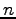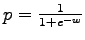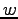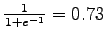Next: 3.3 Multinomial Distribution Up: 3 The Basics Previous: 3.1 Uniform Distribution

## 3.2 Binomial Distribution

We can also model a binomial distribution as an TML KB. If we change the initial coin TML KB from Section 2.1 and add a weight w to the value Heads of attribute Side, our .tml file is updated as follows:

class FlipSequence {
Side Heads 1.0, Tails;
}

Note that if no weight is given to an attribute, the default is a weight of 0.0. An equivalent update to the .tml file is:

class FlipSequence {
Side Heads 1.0, Tails 0.0;
}

we have a binomial distribution withbeing the number of flips (in our case 10) and, whereis the weight of the unit clause (in our case 1). We can verify this by running probabilistic inference:

al -i binomial_coin.tml -o binomial_coin.result -e coin.db -q Side(Flip)


The marginal probabilities for values of Heads returned will be.Next: 3.3 Multinomial Distribution Up: 3 The Basics Previous: 3.1 Uniform Distribution

Chloe Kiddon 2013-04-01